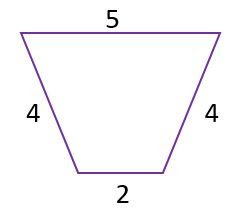## Test Prep Plan - Take a practice test

Take this practice test to check your existing knowledge of the course material. We'll review your answers and create a Test Prep Plan for you based on your results.
How Test Prep Plans work
1
2Based on your results, we'll create a customized Test Prep Plan just for you!
3Study smarter
Study more effectively: skip concepts you already know and focus on what you still need to learn.

# Holt Geometry: Online Textbook Help Final Exam

Free Practice Test Instructions:

Choose your answer to the question and click 'Continue' to see how you did. Then click 'Next Question' to answer the next question. When you have completed the free practice test, click 'View Results' to see your results. Good luck!

#### Question 1 1. In the pictured triangle, ∠A is 98 degrees and ∠B is 12 degrees. If side a is 84 units long, approximately how long is side b?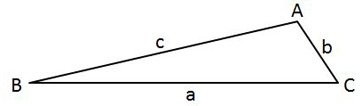#### Question 2 2. In the pictured triangle, ∠A is 137 degrees and ∠B is 28 degrees. If side b is 71 units long, approximately how long is side a?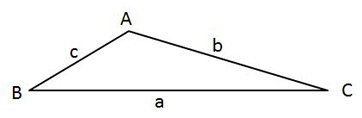#### Question 3 3. What is x?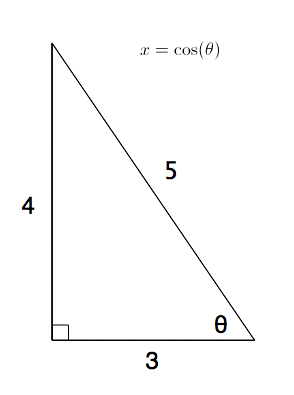#### Question 4 4. What is x?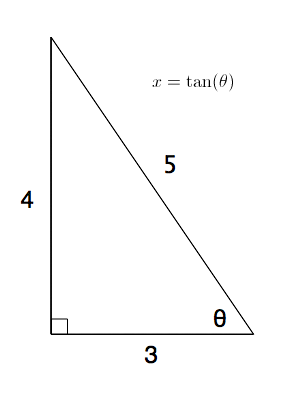#### Question 5 5. Consider the figure below, where line a is parallel to line b: Angle 4 = 12m - 4 Angle 5 = 10m + 20 Calculate the measure of Angle 5.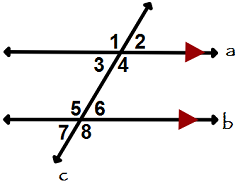#### Question 9 9. Mary claims that the triangles below are similar by AA. Do you agree or disagree with Mary?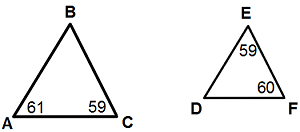#### Question 14 14. Find the perimeter.